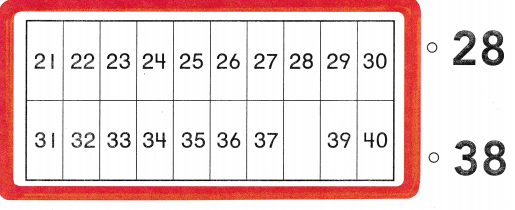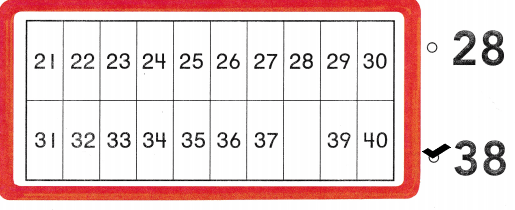# Texas Go Math Kindergarten Lesson 16.1 Answer Key Count to 50 by Ones

Refer to our Texas Go Math Kindergarten Answer Key Pdf to score good marks in the exams. Test yourself by practicing the problems from Texas Go Math Kindergarten Lesson 16.1 Answer Key Count to 50 by Ones.

## Texas Go Math Kindergarten Lesson 16.1 Answer Key Count to 50 by Ones

Essential Question
How does the order of numbers help you count to 50 by ones?

Explore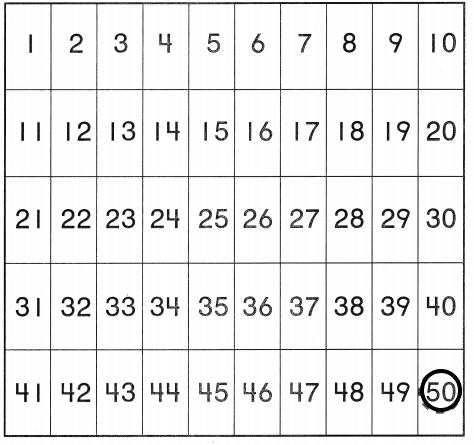Explanation:
I pointed to each number as i counted
I traced the circle around the number 50.

Directions
Point to each number as you count to 50. Trace the circle around the number 50.

Share and Show

Question 1.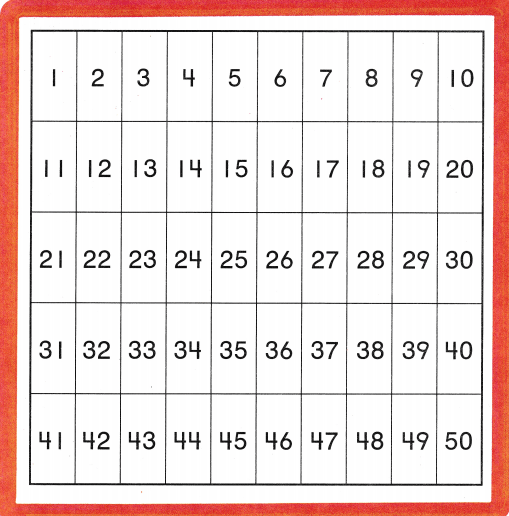Explanation:
I pointed each number as i counted.
I drew a line under the number 50
I drew a circle around the number 15 and counted forward till 50
I colored the box with the number 39 yellow and counted forward till 50
I marked X on the number 20 and counted backward from 20 to 1.

Directions
1. Point to each number as you count to 50. Draw a line under the number 50. Circle the number 15. Begin with 15 and count forward to 50 again. Color the box with the number 39 yellow. Begin with 39 and count forward to 50 again. Mark an X on the number 20. Count backward from 20 to 1.

Question 2.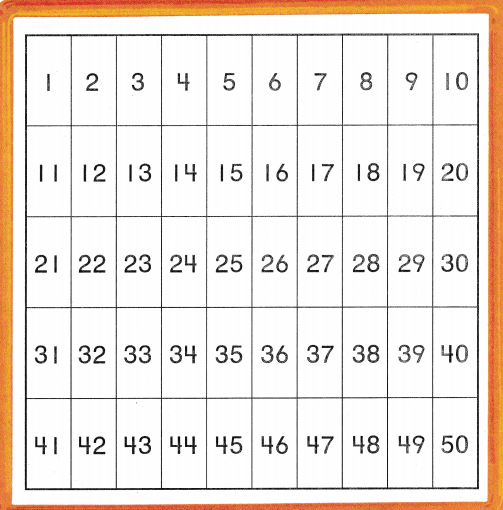Explanation:
I pointed each number as i counted.
I drew a line under the number 50
I colored the box with the number 41 yellow and counted forward till 50
I marked X on the number 20 and counted backward from 20 to 1.

Directions
2. Look away and point to any number. Circle that number. Count forward from that number to 50. Draw a line under the number 50. Find the number that comes right after 40. Color the box with that number yellow. Count forward from that number to 50. Mark an X on number 20. Count backward from 20 to 1.

Home Activity

• Think of a number between 1 and 50 and use clues to help your child guess your number. For example. My number is one more than 25, What is my number? Have your child say the number.

Problem Solving

Question 3.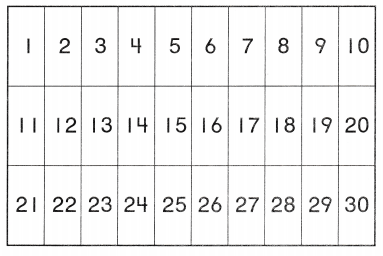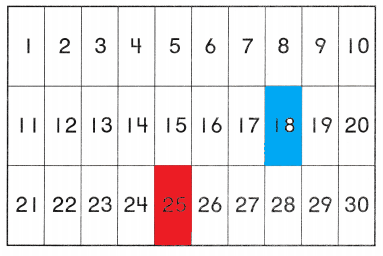Explanation:
The number that comes after 17 is 18. So, i colored it blue
The number that comes before 26 is 25. So, i colored it red.

Question 4.Explanation:
I pointed to each number that i counted
The missing number is 44
So, i checked the number 44.

Directions
3. What number is one more than 17? Use blue to color the box with that number. What number is one less than 26? Use red to color the box with that number. 4. Choose the correct answer. Point to each number as you count. What is the missing number?

### Texas Go Math Kindergarten Lesson 16.1 Homework and Practice

Question 1.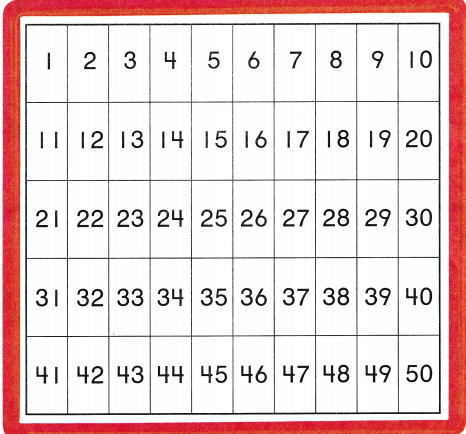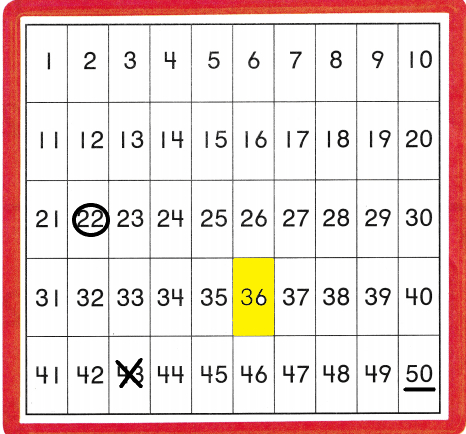Explanation:
I pointed each number as i counted.
I drew a line under the number 50
I colored the box with the number 36 yellow and counted forward till 50
I marked X on the number 43 and counted forward till 50.

Directions
1. Point to each number as you count to 50. Draw a line under the number 50. Circle the number 22. Begin with 22 and count forward to 50. Find the number 36. Color the box with the number 36 yellow. Begin with 36 and count forward to 50. Mark on X on the number right after 42. Count forward from that number to 50.

Texas Test prep

Lesson Check

Question 2.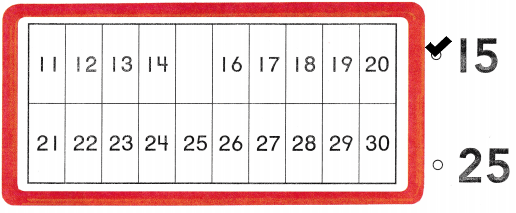Explanation:
I pointed to each number that i counted
The missing number is 15
So, i checked the number 15

Question 3.